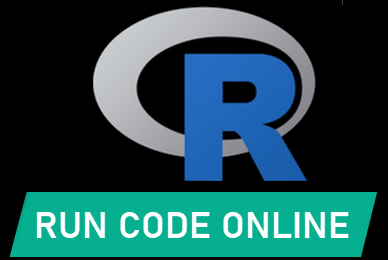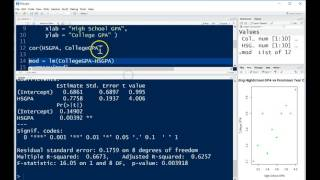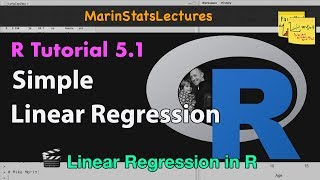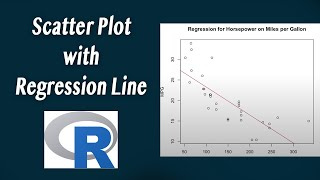R Tutorials & Resources:
Chapter 11: Correlation and Bivariate Regression
 Suggest a Link Alphabetize Page Printer-Friendly List
Watch and Learn
Watch and Learn
Watch and Learn
Watch and Learn
 Creating Map from Correlation Matrix Demo.Demonstrates a powerful visual application of correlation analysis.Tutorial on Bivariate RegressionFrom Katie Ann Jager (11:32)Simple Linear RegressionFrom Mike Marin (5:37)How to Make a Scatterplot in R (with Regression Line)From thatRnerd (5:01)Creating Map from Correlation Matrix Demo.Demonstrates a powerful visual application of correlation analysis.Tutorial on Bivariate RegressionFrom Katie Ann Jager (11:32)Simple Linear RegressionFrom Mike Marin (5:37)Creating Map from Correlation Matrix Demo.Demonstrates a powerful visual application of correlation analysis.Tutorial on Bivariate RegressionFrom Katie Ann Jager (11:32)Creating Map from Correlation Matrix Demo.Demonstrates a powerful visual application of correlation analysis.R Functions and Packages for Political Science Analysis Great tutorials on useful functions and packages.Help & Resources
 Cookbook for R Excellent reference book by Winston ChangHelp & Resources
 R Commander A graphical user interface for the R program.Help & Resources
 R Package Documentation A comprehensive index of R packages and documentation, tools to run code online and embed samples.Help & Resources
 R Functions and Packages for Political Science Analysis Great tutorials on useful functions and packages.Help & Resources
 Cookbook for R Excellent reference book by Winston ChangHelp & Resources
 R Commander A graphical user interface for the R program.Help & Resources
 R Functions and Packages for Political Science Analysis Great tutorials on useful functions and packages.Help & Resources
 Cookbook for R Excellent reference book by Winston ChangHelp & Resources
 R Functions and Packages for Political Science Analysis Great tutorials on useful functions and packages.Help & Resources